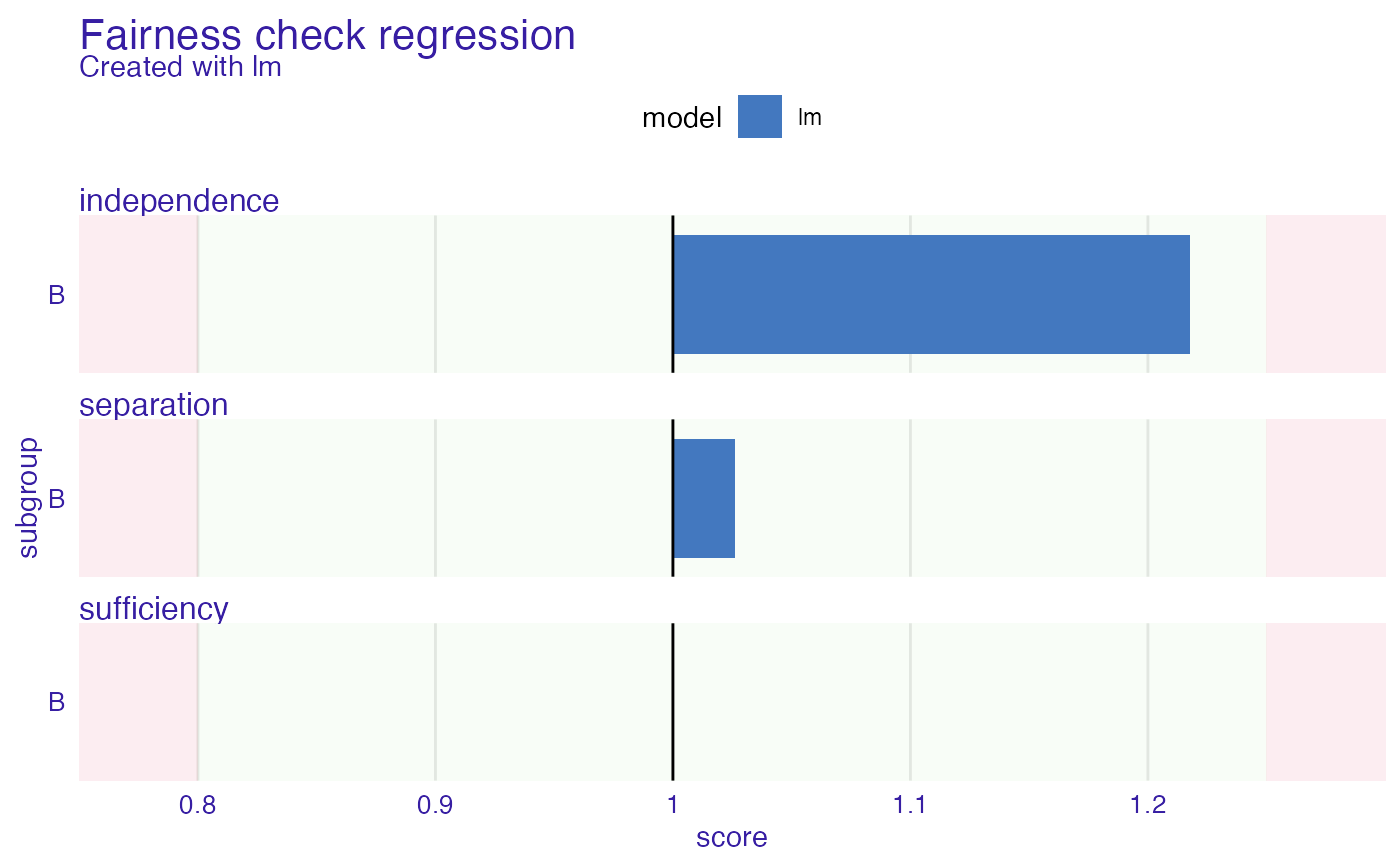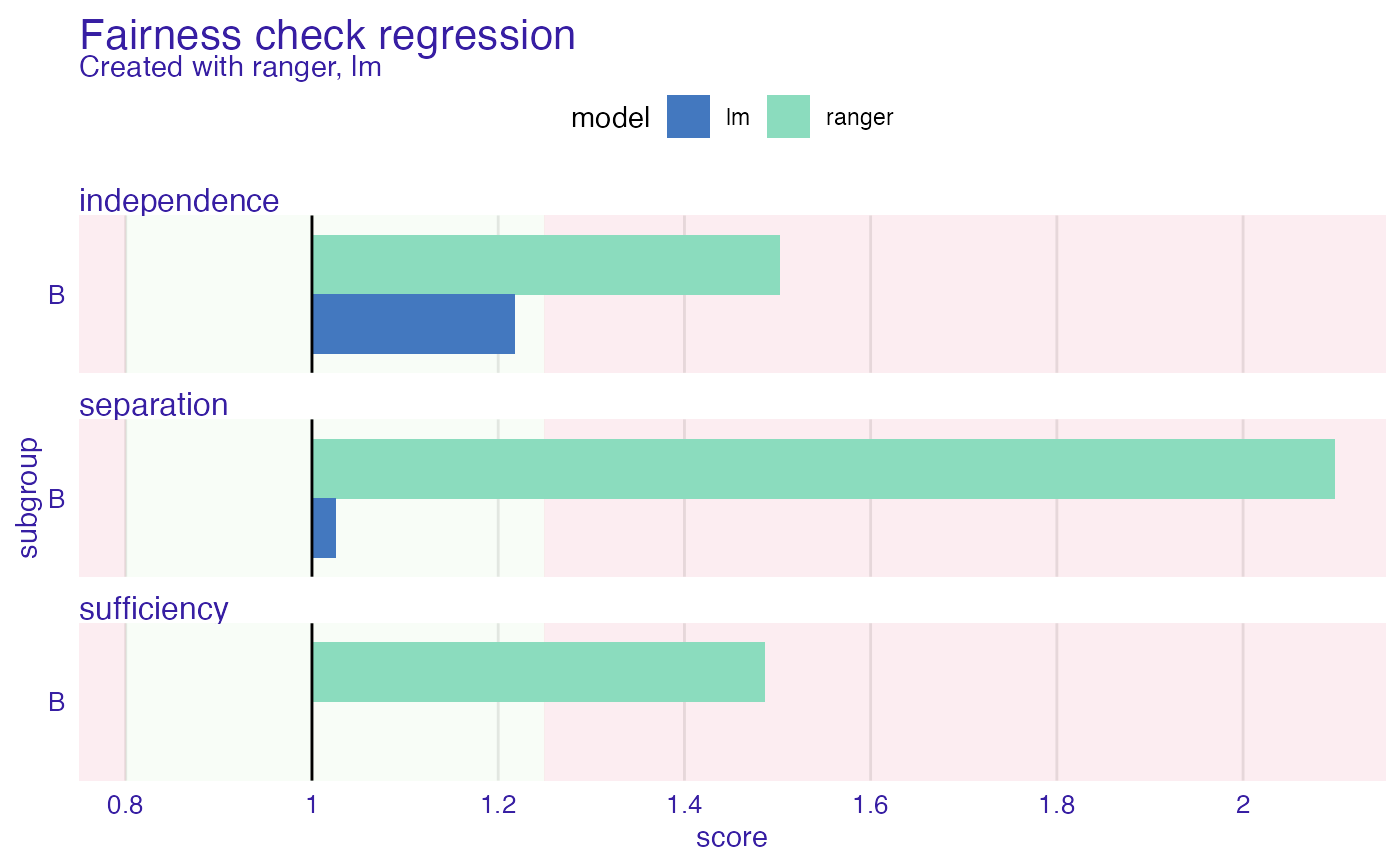This is an experimental approach. Please have it in mind when using it. Fairness_check_regression enables to check fairness in regression models. It uses so-called probabilistic classification to approximate fairness measures. The metrics in use are independence, separation, and sufficiency. The intuition behind this method is that the closer to 1 the metrics are the better. When all metrics are close to 1 then it means that from the perspective of a predictive model there are no meaningful differences between subgroups.

fairness_check_regression(
x,
...,
protected = NULL,
privileged = NULL,
label = NULL,
epsilon = NULL,
verbose = TRUE,
colorize = TRUE
)

Arguments

x object created with explain or of class fairness_regression_object. It can be multiple fairness_objects, multiple explainers, or combination on both, as long as they predict the same data. If at least one fairness_object is provided there is no need to pass protected and privileged parameters. Explainers must be of type regression possibly more objects created with explain and/or objects of class fairness_regression_object factor, protected variable (also called sensitive attribute), containing privileged and unprivileged groups factor/character, one value of protected, denoting subgroup suspected of the most privilege character, vector of labels to be assigned for explainers, default is explainer label. numeric, boundary for fairness checking, lowest/maximal acceptable metric values for unprivileged. Default value is 0.8. logical, whether to print information about creation of fairness object logical, whether to print information in color

Details

Sometimes during metric calculation faze approximation algorithms (logistic regression models) might not coverage properly. This might indicate that the membership to subgroups has strong predictive power.

Steinberg, Daniel & Reid, Alistair & O'Callaghan, Simon. (2020). Fairness Measures for Regression via Probabilistic Classification. - https://arxiv.org/pdf/2001.06089.pdf

Examples

set.seed(123)
data <- data.frame(
x = c(rnorm(500, 500, 100), rnorm(500, 400, 200)),
pop = c(rep("A", 500), rep("B", 500))
)

data$y <- rnorm(length(data$x), 1.5 * data$x, 100) # create model model <- lm(y ~ ., data = data) # create explainer exp <- DALEX::explain(model, data = data, y = data$y)
#> Preparation of a new explainer is initiated
#>   -> model label       :  lm  (  default  )
#>   -> data              :  1000  rows  3  cols
#>   -> target variable   :  1000  values
#>   -> predict function  :  yhat.lm  will be used (  default  )
#>   -> predicted values  :  No value for predict function target column. (  default  )
#>   -> model_info        :  package stats , ver. 4.1.1 , task regression (  default  )
#>   -> predicted values  :  numerical, min =  -269.546 , mean =  681.4906 , max =  1444.562
#>   -> residual function :  difference between y and yhat (  default  )
#>   -> residuals         :  numerical, min =  -302.6659 , mean =  -9.167582e-14 , max =  332.7938
#>   A new explainer has been created!

# create fobject
fobject <- fairness_check_regression(exp, protected = data$pop, privileged = "A") #> Creating fairness regression object #> -> Privileged subgroup : character ( Ok ) #> -> Protected variable : factor ( changed from character ) #> -> Fairness objects : 0 objects #> -> Checking explainers : 1 in total ( compatible ) #> -> Metric calculation : 3/3 metrics calculated for all models #> Fairness regression object created succesfully #> # results fobject #> #> Fairness check regression for models: lm #> #> lm passes 3/3 metrics #> Total loss: 0.243362 #> plot(fobject)# \donttest{ model_ranger <- ranger::ranger(y ~ ., data = data, seed = 123) exp2 <- DALEX::explain(model_ranger, data = data, y = data$y)
#> Preparation of a new explainer is initiated
#>   -> model label       :  ranger  (  default  )
#>   -> data              :  1000  rows  3  cols
#>   -> target variable   :  1000  values
#>   -> predict function  :  yhat.ranger  will be used (  default  )
#>   -> predicted values  :  No value for predict function target column. (  default  )
#>   -> model_info        :  package ranger , ver. 0.13.1 , task regression (  default  )
#>   -> predicted values  :  numerical, min =  210.6774 , mean =  681.3779 , max =  987.8878
#>   -> residual function :  difference between y and yhat (  default  )
#>   -> residuals         :  numerical, min =  -668.0995 , mean =  0.1126996 , max =  629.5469
#>   A new explainer has been created!

fobject <- fairness_check_regression(exp2, fobject)
#> Creating fairness regression object
#> -> Privileged subgroup		: character ( from first fairness object  )
#> -> Protected variable		: factor ( from first fairness object  )
#> -> Fairness objects		: 1 object (  compatible  )
#> -> Checking explainers		: 2 in total (  compatible  )
#> -> Metric calculation		: 3/3 metrics calculated for all models
#>  Fairness regression object created succesfully
#>

# results
fobject
#>
#> Fairness check regression for models: ranger, lm
#>
#> ranger passes 0/3 metrics
#> Total loss:  2.086092
#>
#> lm passes 3/3 metrics
#> Total loss:  0.243362
#>

plot(fobject)# }